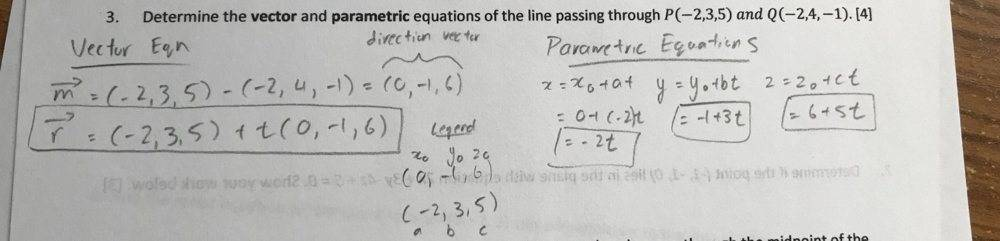# Calculus and Vectors - Vector and Parametric Equations

• ttpp1124

#### ttpp1124

Homework Statement
Calculus and Vectors - Vector and Parametric Equations
I solved it, can someone concur?
Relevant Equations
Not Available-

For the parametric equation, what are the values of ##t## corresponding to the points ##P## and ##Q## that you were given?

Same for the vector equation. ##t = 0## gives you ##P##. What value of ##t## gives you ##Q##?

That's always a good check.

When I sub in 0 for t, I get the values corresponding to the direction vector. That's how I know it's correct

When I sub in 0 for t, I get the values corresponding to the direction vector. That's how I know it's correct

Looks wrong to me!

Looks wrong to me!
how is it wrong?

how is it wrong?
Is that ##x = -2t, \ y = -1 + 3t, \ z = 6 + 5t##?

Is that ##x = -2t, \ y = -1 + 3t, \ z = 6 + 5t##?
Yes

Yes
That's not right. What values of ##t## give you ##P## and ##Q##?

That's not right. What values of ##t## give you ##P## and ##Q##?
Is it the direction vector, 0,-1,6?

Is it the direction vector, 0,-1,6?
The vector equation is correct. That's the direction ##\vec{QP}##.

The vector equation is correct. That's the direction ##\vec{QP}##.
Right, so I think I didn't multiply the t value and do vector addition. When I do multiply, I get r = (-2,3,5)+(0t,-t,6t).
Then I do vector addition by components, so then my parametric equations should be -2+0t, 3-t, and 5+6t

Right, so I think I didn't multiply the t value and do vector addition. When I do multiply, I get r = (-2,3,5)+(0t,-t,6t).
Then I do vector addition by components, so then my parametric equations should be -2+0t, 3-t, and 5+6t
So, what value of ##t## gives you ##Q##?

So, what value of ##t## gives you ##Q##?
-1

•PeroK
-1
My question is, how does the value of t have anything to do with the actual question? They just wanted to know the vector and parametric equations.

My question is, how does the value of t have anything to do with the actual question? They just wanted to know the vector and parametric equations.

Also, are my new parametric equations correct?

My question is, how does the value of t have anything to do with the actual question? They just wanted to know the vector and parametric equations.

Also, are my new parametric equations correct?